﻿ Is the String Theory Still Alive? Exploration of the Mass of Photon and the Origin of MatterPublications are Open
Access in this journal
Article Versions
Export Article
• Normal Style
• MLA Style
• APA Style
• Chicago Style
Research Article
Open Access Peer-reviewed

### Is the String Theory Still Alive? Exploration of the Mass of Photon and the Origin of Matter

Zhonglin BO
International Journal of Physics. 2022, 10(2), 88-92. DOI: 10.12691/ijp-10-2-1
Received January 20, 2022; Revised February 23, 2022; Accepted March 01, 2022

### Abstract

Based on the Maxwell’s electromagnetic theory and Planck’s energy quantum theory, a new theory of energy and mass relation of a photon in wave motion was developed. The theory provided a way of measuring the mass of a photon in wave motion. With innovative incorporation of chemical bond theory, a new theory of photon molecule with π chemical bond and σ chemical bond at extreme conditions was also developed. This theory clearly illustrated the mechanism of mass generation of matter as the proceeding of photon’s polymerization based on the energy conservation. It provided the sound explanation of the generation of photon string and photon membrane as the building block of the matter. It also articulated how a photon to form a stable photon dipole from spin point-charges. It also elucidated the intrinsic nature of electromagnetic waves attributed to the different pole distance of photons.

### 1. Introduction

In 1954 C.N. Yang and R. Mills developed the concept of gauge theory for abelian groups to provide an explanation for strong interactions 1. In 1961 S. Glashow combined the electromagnetic and weak interactions 2. In 1967 S. Weinberg 3 and A. Salam 4 incorporated the Higgs mechanism 5, 6, 7 into Glashow's electroweak interaction to give its modern form.

The Higgs mechanism is believed to generate the masses of all the elementary particles in the Standard Model. This includes the masses of the W, Z bosons, and the masses of the fermions, such as quarks and leptons.

Interactions in physics are the ways that particles exert force to other particles. At a macroscopic level, electromagnetism allows particles to interact with one another via electric, magnetic fields, while gravitation allows particles with mass to attract one another in accordance with Einstein's general relativity. The Standard Model explains such forces as resulting from matter particles exchanging other particles, generally referred to as force mediating particles. When a force-mediating particle is exchanged, the effect at a macroscopic level is equivalent to a force influencing both of them, and the particle is therefore said to have mediated that force 8.

The Standard Model provides a unified description of three of the fundamental forces of nature: electromagnetic force, the strong and weak nuclear forces. Despite its remarkable success in explaining a wide range of physical phenomena, the standard model cannot be a complete description of reality. This is because the standard model failed to incorporate the force of gravity and because of problems such as the hierarchy problem and the inability to explain the structure of fermion masses.

String theory is a modern theory that attempts to answer these questions and to illustrate many others. The starting point for string theory is the idea that the point-like particles can also be modeled as one-dimensional objects called strings 9. String theory describes how strings propagate through space and interact with each other. In some version of string theory, there is only one kind of string, which may look like a small loop 10 or section of ordinary string, and it can vibrate in different ways. On distance scales larger than the string scale, a string will look just like an ordinary particle, with its mass, charge, and other properties determined by the vibrational state of the string.

In string theory and other related theories, a brane is a physical object that describes the notion of a point particle to higher dimensions. For instance, a point particle can be viewed as a brane of dimension zero, while a string can be viewed as a brane of dimension one. It is also possible to consider higher-dimensional branes 11. In dimension p, these are called p-branes. The word brane comes from the word “membrane” which refers to a two-dimensional brane.

The String theory was a broad and varied subject that tries to address a lot of deep questions of fundamental physics. It had been used to construct a variety of models of particle physics going beyond the standard model. Because string theory potentially provides a unified description of gravity and particle physics, it was a most potential candidate for a theory of everything, a self-contained mathematical model that describes all fundamental forces and forms of matter.

Starting with ten or eleven dimensional spacetime of string or M-theory 12, physicists postulated a shape for the extra dimensions. By choosing this shape appropriately, they could construct models roughly similar to the standard model of particle physics, together with additional undiscovered particles 13. One popular way of deriving realistic physics from string theory was to start with the heterotic theory in ten dimensions and assume that the six extra dimensions of spacetime were shaped like a six-dimensional Calabi-Yau manifold. Such compactifications offered many ways of extracting realistic physics from string theory. Other similar methods were used to construct realistic or semi-realistic models of our four-dimensional world based on M theory 14.

Up to now, neither Standard Model nor the String theory can elucidate how the mass is generated, how the matter is generated. This paper tried to develop a new theory to illustrate the generation mechanism for both the photon and the matter.

### 2. Theory Deduction

According to Maxwell’s equations 15, the electromagnetic wave equation of plane light waves in one dimension is expressed as Equation 1, the solution to the derivative equation shall be a cosine function as Equation 2, where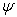is the wave function,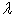is the wave length,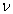is the frequency,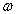is the angular frequency,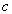is the wave speed: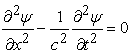(1)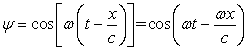(2)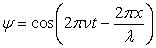or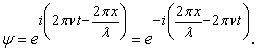If is applied to state that the energy of waves is quantized, where h is Plank constant, ħ is the reduced Plank constant, E is the energy, and p is the momentum: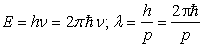then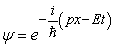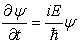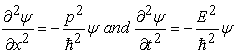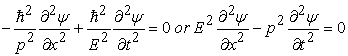(3)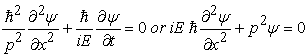(4)

If we substitute the p with mc in Equation 3 and 4, then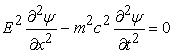(5)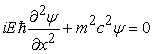(6)

If a wave equation is described in three dimensions, the equation 5, 6 shall be as: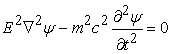(7)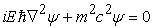(8)

The Equation 5, 6, 7 and 8 describe the energy and mass relation of the photon during wave motion. And after we compare Equation 1 with Equation 3, we shall derivate: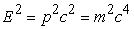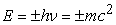(9)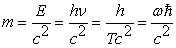(10)

Therefore the equation 10 shall tell us how to measure the mass of a photon in wave motion, even though the static mass of a photon is zero.

### 3. Photon Bonding

We all know that photon and anti-photon or gluon are electric dipoles 16 or magnetic dipoles. We suppose their spin modes shall be described as Figure 1, arrow head as the positive electric point-charge, small circle as the negative electric point-charge, the big circle as the spin trajectory of the dipole without considering the motion forward intentionally. When two spinning photons approach close enough, they shall take two different ways to bind together. When the two spin axes are parallel, one with counter clockwise direction, the other with clockwise direction. The magnetic poles of each photon are induced as different attractive pole pairs (N-S), there are constant attractive magnetic forces at the spin centers of two photons both above and beneath the spin planes. The repulsive electric force will follow as sinusoidal function between two positive electric poles and two negative electric poles during 2π period, the two photons will be bonded in an oscillating mode if the two vectors are with zero initial phase difference; While if the two vectors are with π initial phase difference, the two photons shall be annihilated as illustrated as Figure 2. If the initial vector phase difference is π/2, the repulsive and attractive electric forces are constant, the total forces between two photons are balanced between magnetic attractive force and repulsive electric force. The two photons are bonded by net magnetic force as photon molecule with shoulder to shoulder, π chemical bond, represented as Figure 3.

• Figure 1. The spin modes of photon and gluon
• Figure 2. The photons approach in different initial phase differences
• Figure 3. The photon molecule with π bond

When the two spin planes are on the same axis, parallel to each other, but with same spin direction, either counter clockwise or clockwise. Same as shoulder to shoulder π bond, all the magnetic poles of each photon are induced as different attractive pole pairs(N-S), but just between the space of the two spin parallel planes. When the two photons’ relative positions are synchronized, all the forces no matter magnetic or electric shall be constant. Since there are same distances between two positive poles and two negative poles, while the distance of the magnetic poles induced by two spin dipoles is almost zero. So the total attractive magnetic force shall be easily balanced with the total repulsive electric forces at some distance. The two photons are bonded as photon molecule with head to head, σ chemical bond represented as Figure 4. After comparing with two bonds of the photons, we think photon molecule with σ bond shall be more stable than photon molecule with π bond.

• Figure 4. The photon molecule with σ bond

Furthermore, if hundreds of, thousands of, millions of, even more photons are aligned in a line with π bond approach, all the photons on the line are bonded together as a line with attractive magnetic force as the force of chemical bond, the polymetric photon bond is formed as Figure 5. Of course, there shall be another polymetric photon bond based on σ bond represented as Figure 6.

• Figure 5. The photons with polymetric π bond
• Figure 6. The photons with polymetric σ bond

Again, we believe the polymetric photons with σ bond shall be more stable than the polymetric photons with π bond. We may call these polymetric photons as photon polymer. In order to make it simple, hereafter we will refer to this photon polymer only with σ bond for further discussion.

### 4. Discussion

4.1. The Mass Generation

With the photons form molecule or photon polymer, the photon polymer or each photon participating polymerization shall obtain the bonding energy or potential energy, according to energy conservation law, each photon or photon polymer shall lose kinetic energy and momentum; At the condition of constant motion mass, each photon or polymer shall decrease motion speed. According to Einstein’s special relativity as described as Equation 11, then each photon or photon polymer shall increase its static mass. Then the photon polymer shall become heavier and heavier due to energy conservation as polymerization continues. The polymerization of photons shall be the mechanism of static mass generation of the matter.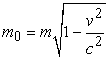(11)

According to the Higgs mechanism 17, it provides a general framework to explain the observed masses of the W± and Z gauge bosons by means of charged and neutral Goldstone bosons that end up as the longitudinal components of the gauge bosons. These Goldstone bosons are generated by the underlying dynamics of electroweak symmetry breaking (EWSB). However, the fundamental dynamics of the electroweak symmetry breaking are unknown.

While the String Theory 18 describes how strings propagate through space and interact with each other. In a given version of string theory, point-like particles can be modeled as one-dimensional objects, strings which may look like a small loop or segment of ordinary string, and it can vibrate in different ways. On distance scales larger than the string scale, a string will look just like an ordinary particle, with its mass, charge, and other properties determined by the vibrational state of the string. But how the vibrating state generating the particle mass is also unknow.

4.2. The Matter Generation

If we treat this linear polymer as a one-dimensional photon fiber or photon string as Figure 7, the string will form a loop because the two ends of the string are with different magnetic poles, this may be the loop of the String Theory. If two photon strings approach in parallel way, but with different magnetic poles closing together (to turn around the head and tail), a two-dimensional photon membrane will form as Figure 8, with balanced magnetic force and electric force. The sting theory may have described the properties of this membrane. Of course the photon strings can form three-dimensional laminate in similar way.

• Figure 8. The photon membrane

The theory that the material is generated from the polymerization of photons can explain the phenomenon of charging by friction, different kind of properties of static electricity, and different static charge separation such as contact-induced charge separation, pressure-induced charge separation, heat-induced charge separation and charge-induced charge separation. Because all the matter is made of this photon polymer including the starting building blocks of matter, protons, electrons and neutrons.

When we re-configured the new structure of Proton, Electron and Neutron in another paper, we once raised a question about what the super insulation material is made of. Now we find the answer, it must be the photon polymer. This material generation mechanism shall strongly support our theory of new Atom Configuration and prove the Higgs particle as the insulation material fused from the empty balls 19. Since the mechanic strength of the material is very strange, even in the high energy Large Hadron Collider, fission or fusion of the material can happen, but it can’t be destroyed. From this perspective, it demonstrates that the binding energy of the photon polymer between the photons is much high. In another word, the polymerization of the photons will not happen at normal conditions. It would happen only at high energy conditions similar to Large Hadron Collider or higher.

If we consider the characteristics of electric charge, spin and the mass almost zero of electron neutrino and electron anti-neutrino, The electron neutrinos are most probably the photon molecular, because they are created through radioactive decay or natural such as those that take place in the core of a at high energy conditions.

If the photon molecular or polymer properties are considered, this theory shall provide some restriction conditions to String Theory, maybe the eleven-dimensional space or six-dimensional Calabi-Yau space will be reduced to the realistic four-dimensional spacetime.

4.3. The Photon Dipole Formation

Once we get the answer about what material the matter is made from. Naturally the next question will be how the photon can be stable without annihilation. In order to articulate this, and to make it simple, we suppose the positive point-charge as a small ball with positive charge while the negative point-charge as a small ball with negative charge as Figure 9. Both point-charges are spinning with same speed but with different spin direction, one in clockwise direction the other in counter-clockwise direction. When these point-charges approach closely together. There is attractive electric force between two point-charges to pull them together; while due to different spin direction, the induced magnetic forces with repulsive same magnetic poles (N-N and S-S). both in front of the paper or behind the paper. Immediately the attractive electric force shall be balanced by the repulsive magnetic forces. The stable photon dipole is finally formed with a constant distance between a positive point-charge and a negative point-charge, pole distance (d) of a photon. The spin photon dipole actually moves with light speed c as an electromagnetic wave of an electric vector.

• Figure 9. The formation of Photon dipole

The pole distance (d) of a photon shall be determined by the spin speed of the point-charges. The higher spin speed of the point-charges, the shorter the pole distance(d) between photon di-poles will be balanced. The wave length(λ) of a light (electromagnetic wave) shall be determined by the pole distance (d) of a photon. In another word, the different electromagnetic waves are just attributed to the different photons with different pole distances(d) of photons (λ=2πc/ω =πdc/(ωr), mid-point of the dipole as spin center, c as the light speed, ω as the angular frequency of the spin photon, r as the half pole distance of the photon).

The formation theory of photon dipole shall illustrate the intrinsic nature of Electromagnetic spectrum from ionizing radiation to radio waves, the shorter wave length, the shorter pole distance of the photon, the shorter period T, the higher spin speed of the photon, the higher frequency and the higher energy level of the photon, because the wave speed is at constant c.

Finally we suppose the mass of photon is composed of equal mass (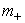=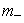= m/2) of positive point-change (PPC)and negative point-charge(NPC), then according to Einstein’s energy equation of photon, E=mc2, Ek as photon’s kinetic energy, Ep as photon’s potential energy or the point-charge binding energy.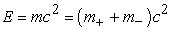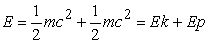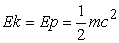(12)

According to equation 12, we can deduce that the binding energy of the photon between positive point-charge and negative point-charge is same as photon’s kinetic energy, and we can deduce again that the molecularization or polymerization of photons shall require similar level of potential energy to bind the photons together. It seems only the high energy level in the core of stars or the higher energy level described by The Big Bang Theory 20 will meet such extremely rigorous requirements.

### 5. Conclusion

Based on Maxwell’s electromagnetic theory of photon’s plane wave and , we developed a new relation of energy and mass of a photon in wave motion and developed a way to measure the motion mass of photon in wave motion. After innovatively incorporating the chemical bond theory, we developed a new theory that the photons at some extreme conditions will form photon molecule with both π bond and σ bond, and the photons can polymerize with polymetric π bond and polymetric σ bond to form photon polymer at some extreme conditions. The mass of the matter is generated and attributed to photon’s polymerization according to the conservation law of energy, and the mass equation of Einstein’s special relativity. Compared with the Higgs mechanism of particle mass generation and particle mass attributed to string vibration states by String theory, our theory of mass generation due to photon’s molecularization or polymerization is much simpler and more straight forward based on classic physics and chemistry.

Based on the exploration of dipole formation by spin point-charges, we clearly elucidated the intrinsic nature of Electromagnetic spectrum attributed to different pole distances of photons with different spin speeds.

We concluded all the matter is made of photon polymer, which is self consistent with our new atomic configuration and which can explain all the properties of static electricity of the matter. Though finally we illustrated the mechanism of the formation of a stable photon dipole through the motion and force analysis of the point-charges, where the electric positive point-charge and negative point-charge come from is still an intrinsic question we need to further explore to understand the origin of the matter and nature.

### References

  C.N. Yang, R. Mills, “Conservation of Isotopic Spin and Isotopic Gauge Invariance”. Physical Review. 96 (1): p191-195(1954). In article View Article  S.L. Glashow, “Partial-symmetries of weak interactions”. Nuclear Physics. 22(4): p579-588(1961). In article View Article  S. Weinberg, “A Model of Leptons”. Physical Review Letters. 19(21): p1264-1266(1967). In article View Article  A. Salam (1968). N. Svartholm (ed.). Elementary Particle Physics: Relativistic Groups and Analyticity. Eighth Nobel Symposium. Stockholm: Almquvist and Wiksell. p367. In article  F. Englert, R. Brout, “Broken Symmetry and the Mass of Gauge Vector Mesons”. Physical Review Letters. 13(9): p321-323(1964). In article View Article  P.W. Higgs, “Broken Symmetries and the Masses of Gauge Bosons”. Physical Review Letters. 13 (16): p508-509(1964). In article View Article  G.S. Guralnik, C.R. Hagen, T.W.B. Kibble, ”Global Conservation Laws and Massless Particles”. Physical Review Letters. 13(20): p585-587 (1964). In article View Article  J. Gregg, “Exchange Forces in Particle Physics”. Foundations of Physics. 51(1): p13(2021). In article View Article  K. Becker, M. Becker, J. Schwarz, String theory and M-theory: A modern introduction. Cambridge University Press. ISBN 978-0-521-86069-7, 2007. In article  B. Zwiebach, A First Course in String Theory. Cambridge University Press. ISBN 978-0-521-88032-9, 2009. In article View Article  PubMed  P. Aspinwall, T. Bridgeland, A. Craw, M. Douglas, M. Gross, A. Kapustin, G. Moore, G. Segal, B. Szendröi, P.M.H. Wilson, Dirichlet Branes and Mirror Symmetry. American Mathematical Society. ISBN 978-0-8218-3848-8 p13(2009). In article  M. Duff, “The theory formerly known as strings”. Scientific American. 278(2): p64-69(1998) In article View Article  P. Candelas, G. Horowitz, A. Strominger, E. Witten, “Vacuum configurations for superstrings”. Nuclear Physics B. 258: p46-74 (1985). In article View Article  S.T. Yau, S. Nadis, (2010). The Shape of Inner Space: String Theory and the Geometry of the Universe's Hidden Dimensions. Basic Books. ISBN 978-0-465-02023-2. In article  R.A. Serway, J.W. Jewett, Physics for Scientists and Engineers with Modern Physics, Philadelphia: Saunders College Pub., p1267-1326, c1983. In article  Wikipedia, “Standard Model”, https://en.wikipedia.org/wiki/Standard_Model. In article  P.W. Higgs, “Broken symmetries, massless particles and gauge fields”. Phys. Rev. Lett. 12(2), p132-133 (1964). In article View Article  Wikipedia, “String Theory”, https://en.wikipedia.org/wiki/String_theory. In article  Zhonglin Bo, “New Atom Configuration Based on Proton and Electron as Electric Monopole Pairs and Exploration of Quarks and Higgs Particle.” International Journal of Physics, 9(6): p269-274(2021). In article  Wikipedia, “Big Bang”, https://en.wikipedia.org/wiki/Big_Bang. In articleThis work is licensed under a Creative Commons Attribution 4.0 International License. To view a copy of this license, visit http://creativecommons.org/licenses/by/4.0/